Wednesday , May 18 2022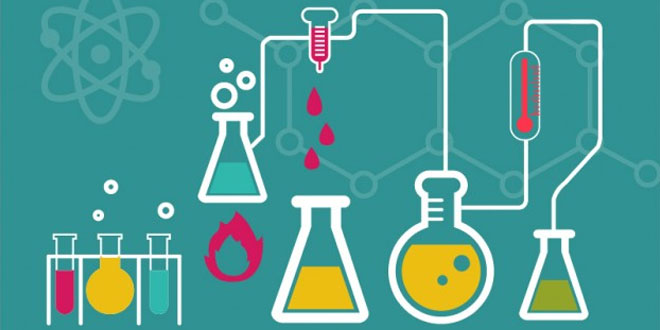# Chemical Reaction & Equations: 10 Science

#### Question: When potassium iodide solution is added to a solution of lead (II) nitrate in a test tube, a precipitate is formed. (i) What is the colour of this precipitate? (ii) Name the compound precipitated. (iii) Write a balanced chemical equation for this reaction. (iv) What type of reaction is this?

(i) Yellow
(ii)
(iii) 2KI + Pb(NO3)2    →     PbI2↓   + 2KNO3
(iv)
This is a double displacement reaction. It can also be called a precipitation reaction.

#### Question: “A solution of potassium chloride when mixed with silver nitrate solution, an insoluble white substance is formed”. (i) Translate the above statement into a chemical equation. (ii) State two types of this reaction.

Answer: (i) KCl (aq)  + AgNO3(aq) → AgCl(s) ↓   +   KNO3(aq)

(ii)
(a) Double displacement reaction.
(b) Precipitation reaction.

#### Question: “Barium chloride reacts with aluminium sulphate to give aluminium chloride and a precipitate of barium sulphate”. (i) Translate the above statement into a chemical equation. (ii) State two types in which this reaction can be classified.

Answer: (i) 3BaCl2(aq) + Al2(SO4)(aq) → 2AlCl3(aq) + 3BaSO4(s) ↓ (White ppt.)
(ii)
(a) Double displacement reaction.
(b) Precipitation reaction.

#### Question: An aluminium can was used to store ferrous sulphate solution. It is observed that in few days holes appeared in the can. Explain the observation and write chemical equation to support your answer.

Answer: Aluminium beings more reactive displaces ferrous ions from ferrous sulphate solution and this result in the appearance of holes in the aluminium can.
2Al (s) + 3FeSO4(aq) → Al2(SO4)(aq) + 3Fe(s)

#### Question: (1) Why is combustion reaction an oxidation reaction? (2) How will you test whether the gas evolved in a reaction is hydrogen?

1. Combustion reaction is an oxidation reaction because it is always carried in the presence of air or oxygen.
CH4(g) + 2O2(g) → CO2(g) + 2H2O(l)
2. Bring a burning matchstick close to the mouth of the tube from which hydrogen gas escapes. The gas will immediately catch fire and this will be accompanied by pop sound.

#### Question: What is the colour of FeSO4. 7H2O crystals? How does this colour change upon heating? Give balanced chemical equation for the change.

Answer FeSO4. 7H2O is green in colour and loses water of crystallization when it is heat. It is then decomposed to Fe2O3(brown colored), SOand SO3.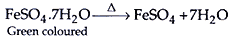2FeSO4(s) → Fe2O3(s) + SO2(g) + SO3(g)

#### (i) Silver bromide on exposure to sunlight decomposes into silver and bromine. (ii) Sodium metal reacts with water to form sodium hydroxide and hydrogen gas.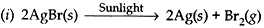(ii) 2Na(s) + 2H2O(l) → 2NaOH(aq) + H2(g)

#### (i) Hydrogen sulphide reacts with sulphur dioxide to form sulphur and water. (ii) Methane on burning combines with oxygen to produce carbon dioxide and water.

Answer: H2S + SO2 → S + H2O
CH+ O2 → CO+ H2O

#### Question: The smell and test of food containing fats and oils changes after sometime. Why does this happen? Name the process. List two ways which help to slow down the above mentioned process.

Answer: Fats and oil get oxidized / they become rancid.
Process: Rancidity.
Keeping food in airtight containers / Refrigeration / Flushing nitrogen in packets of chips, etc.

#### Question: Identify the type of reaction from the following equations: (1) CH4+ 2O2  → CO2 + 2H2O (2)Pb(NO3)2 + 2KI → PbI2 + 2KNO3 (3) CaO + H2O → Ca(OH)2 (4) CuSO4 + Zn → ZnSo4 + Cu

1. Combustion reaction.
2. Double displacement reaction.
3. Combination reaction.
4. Displacement reaction.

#### Question: What is rancidity? Mention any two ways by which rancidity can be prevented.

Answer: The process in which taste and smell of food gets spoiled is called rancidity. It happens due to oxidation.
Prevention from rancidity:

1. Antioxidants are added to fatty acids to prevent oxidation, e.g. chips are packed in presence of nitrogen gas which prevents spoilage by oxidation.
2. Food should be kept in airtight container in refrigerator.

#### Question: The following diagram displays a chemical reaction. Observe carefully and answer the following questions: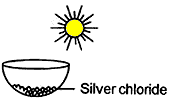(a) Identify the type of chemical reaction that will take place and define it. How will the colour of the salt change?
Write the chemical equation of the reaction that takes place.
(c) Mention one commercial use of this salt.

Answer: (a) Photochemical decomposition reaction: Those reactions in which a compound breaks down into simple substances in presence of light are called photochemical decomposition reaction. The colour of salt will change from white to grey.(c) Silver chloride is used in photography.

#### Question: Study the reactions given below. State which of the following chemical reactions will take place or not, giving suitable reason for each:

1. Zn(s) + CuSO4 (aq) → ZnSO4 (aq) + Cu(s)
2. Fe(s) + ZnSO4 (aq) → FeSO4 (aq) + Zn(s)
3. Zn(s) + FeSO4 (aq) → ZnSO4 (aq) + Fe(s)

1. Zinc is more reactive than copper; so it will displace copper from CuSO4. This reaction will take place.
2. Iron is less reactive than zinc, so it cannot displace zinc from zinc sulphate. The reaction will not take place.
3. Zinc is more reactive than iron, it will displace iron from FeSO4. The reaction will take place.

#### Question: Write the chemical equation of the reaction with an example each in which the following changes have taken place: (1) change in colour. (2) change in temperature. (3) formation of precipitate.

1. Change in colour: Reaction between lead nitrate solution and potassium iodide solution. Change in colour is from colorless to yellow.
Pb(NO3)2(aq) + 2KI(aq) → PbI2(s)+ 2KNO3(aq)
2. Change in temperature: Action of dil. sulphuric acid on zinc.
Zn(s) + H2SO4(aq) → ZnSO4(aq) + H2
3. Formation of precipitate: Action of barium chloride on sodium sulphate.
BaCl2(aq) + Na2SO4(aq) → BaSO4(s) + 2NaCl(aq)

#### (a) Write one Example for each of the decomposition reactions carried out with the help of: (i) electricity (ii) heat (iii) sunlight Give balanced chemical equations in each case. (b) Which of the following statement is correct and why? Copper can displace silver from the solution of silver nitrate and silver can displace copper from the solution of copper sulphate.(b) Copper can displace silver from silver nitrate solution because it is more reactive than silver.
Cu(s) + 2AgNO3(aq) → Cu(NO3)2(aq) + 2Ag(s)

#### Question: Balance the following chemical equations: (1). Al + CuCl2→ AlCl3 + Cu (2) PbO + HCl → PbCl2 + H2O (3) NH3+ O2 → NO + H2O

1. 2Al + 3CuCl2 → 2AlCl3 + 3Cu
2. PbO + 2HCl → PbCl2 + H2O
3. 4NH3+ 5O→ 4NO + 6H2O

#### Question: What is meant by balanced chemical equation? Why chemical equations are balanced? Balanced the chemical equation given below: Al2O3 + NaOH → NaAlO2 + H2O

1. A chemical equation in which the numbers of atoms of each type involved are the same on the reactants and products is called balanced chemical equation.
2. To justify the law of conservation of mass in a reaction, a chemical equation is to be balanced.
3. Al2O3 + 2NaOH → 2NaAlO+ H2O

#### Question: Observer the following diagram and answer the questions that follow: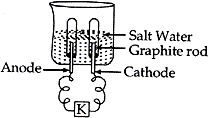#### (1). Identify the gases evolved at anode and cathode. (2). Why are the amounts of gases collected in the two test tubes are of not the same volume? (3). What type of reaction is this? (4). Why should we use salt water?

1. (a) At anode, oxygen gas is evolved.
(b) At cathode, hydrogen gas is evolved.
2. The amounts of gases collected in the two test tubes are of not the same volume because in water, hydrogen and oxygen are present in the ration of 2:1 by mass.
3. This reaction is decomposition reaction.
4. To conduct electricity through it.

#### Question: Balance the following chemical equation and write the chemical name of the products obtained (except water) in the form of compound in each.

1. HNO3 + Ca(OH)2 → Ca(NO3)2 + H2O
2. Al + CuCl2 → AlCl3 + Cu
3. NaOH + H2SO4 → Na2SO4 + H2O

1. 2HNO3 + Ca(OH)2 → Ca(NO3)2 + 2H2O
2. 2Al + 3CuCl2 → 2AlCl3 + 3Cu
3. 2NaOH + H2SO4 → Na2SO4 + 2H2O

#### Question: What is meant by balanced chemical equation? Why chemical equation given below. Al2O3 + NaOH → NaAlO2 + H2O

1. A chemical equation in which the number of reactants on left hand side are equal to the number of products on right hand side is called a balanced chemical equation.
2. Chemical equations are balanced to satisfy the law of conservation of mass.
3. Al2O3 + 2NaOH → 2NaAlO2 + H2O

#### Question: Given differences between the displacement and double displacement reactions.

1. In these reactions, more active element displaces less active element from its salt solution.
2. These reactions are usually slow and take longer time for their completion.
3. During these reactions usually change of colour takes place. e.g.,
Fe(s) + CuSO4 (aq) → FeSO4 (aq) + Cu(s)

Double displacement reaction:

1. In these reactions, there is an exchange of ions between two reactants.
2. These reactions are usually fast and take place instantaneously.
3. During these reactions usually precipitates are formed. e.g.,
AgNO3 (aq) + NaCl (aq) → AgCl(s) ↓ + NaNO3 (aq)

#### Question: A, B and C are three elements which undergo chemical reactions according to the following  equations: A2O3 + 2B → B2O3 + 2A 3CSO4 + 2B → B2(SO4)3 + 3C 3CO + 2A → A2O3 + 3C Answer the following questions with reactions: (1) Which element is the most reactive? (2) Which element is the least reactive? (3) What is the type of reactions listed above?

1. B
B displaced both A and C from their solution.
2. C
C is displaced by both A and B.
3. Displacement reactions.

#### Question: (1) Give an example for a combination reaction which is exothermic. (b) Identify the oxidizing agent, reducing agent in the following reaction: H2S + Cl2 → 2HCl + S (c) Name the phenomenon due to which the taste and smell of oily food changes when kept for a long time in open. Suggest one method to prevent it.

1. 2H2(g) + O2(g) → 2H2O(l) + Heat
2. Oxidizing agent – Cl2
Reducing agent – H2S
3. Rancidity, keeping food in airtight containers

#### Question: A reddish-brown colored metal, used in electrical wires, when powdered and heated strongly in an open china dish, its colour turns black. When hydrogen gas is passed over this black substance, it regains its original colour. Based on the above information, answer the following questions: (i) Name the metal and the black colored substance formed. (ii)Write balanced chemical equations for both the reactions.

(i) Metal is copper
Black colored substance is copper oxide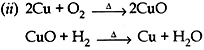#### Question: A small amount of quicklime is added to water in a beaker. (1) Name and define the type of reaction that has taken place. (2) Write balanced chemical equation for the above reaction. Write the chemical name of product obtained. (3) State two observations that you will make in the reaction.

1. It is combination reaction. It is defined as a reaction in which a single product is formed from two or more reactants.
2. CaO(s) + H2O(l) → Ca(OH)2(aq)
(Calcium hydroxide)
3. Calcium oxide:
(i) reacts vigorously with water.
(ii) releasing a large amount of heat.

#### Question: What is redox reaction? Identify the substance oxidized and the substance reduced in the following reactions: (i) 2PbO + C → Pb + CO2 (ii) MnO2 + 4HCl → MnCl2 + 2H2O + Cl2

Answer: Those reactions in which both oxidation and reduction occur simultaneously are called redox reactions.
(i) PbO is reduced, C is oxidized.
(ii) HCl is oxidized, MnO2 is reduced.